# 酱油产品

•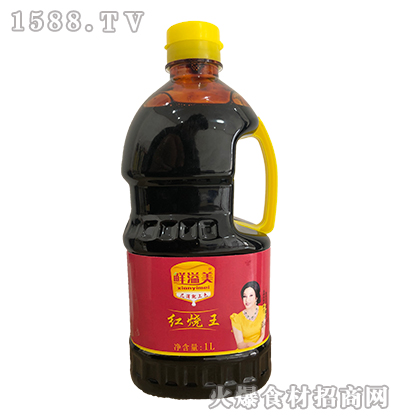我要代理
•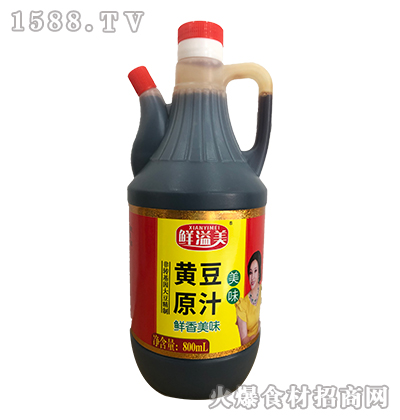我要代理
•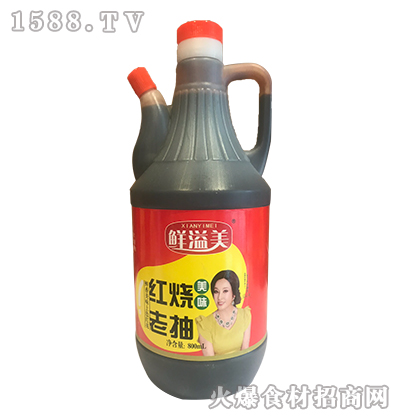我要代理
•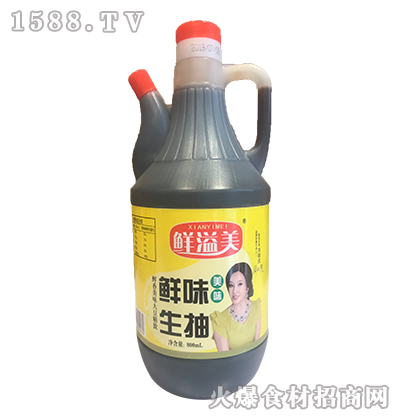我要代理
•我要代理
•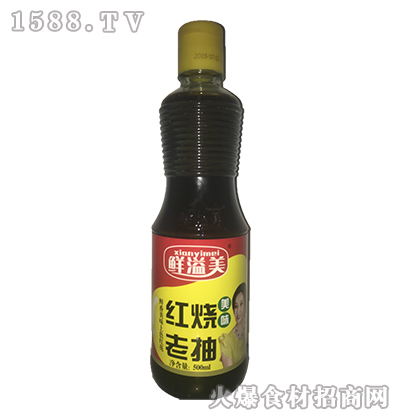我要代理
•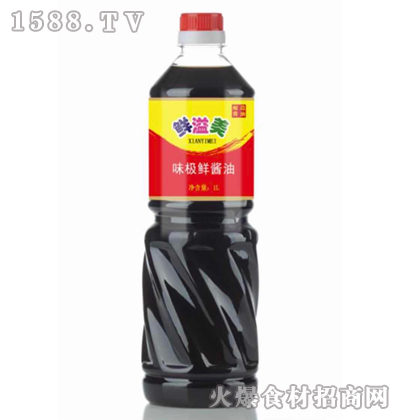我要代理
•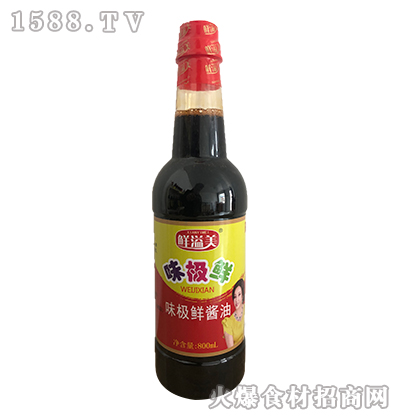我要代理
•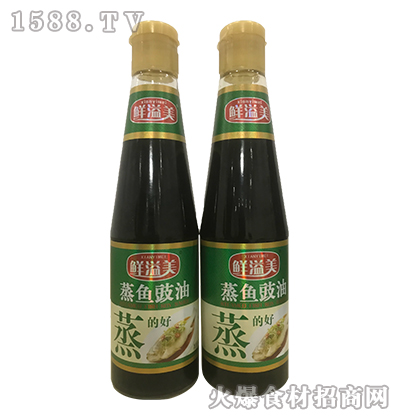我要代理
•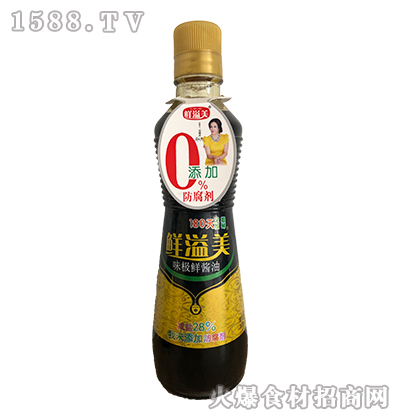我要代理
•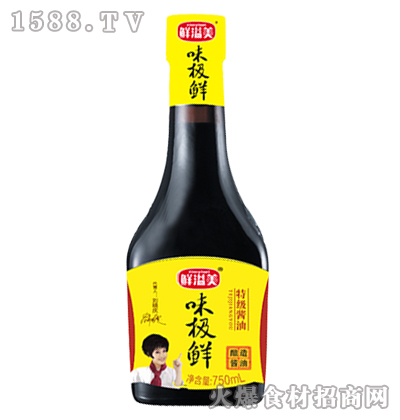我要代理
•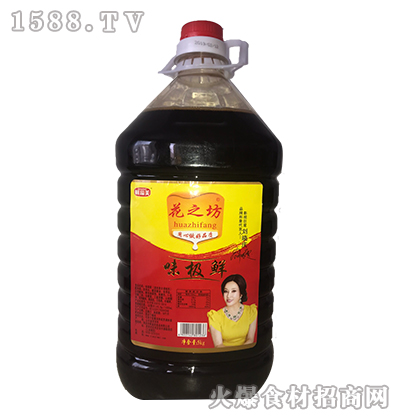我要代理
•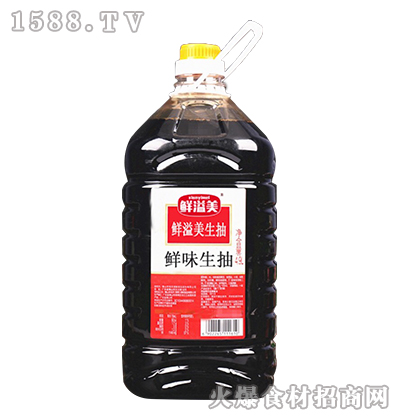我要代理
•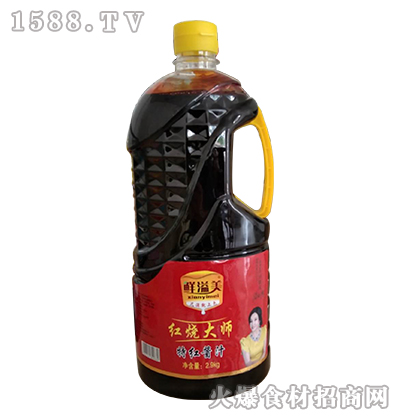我要代理
•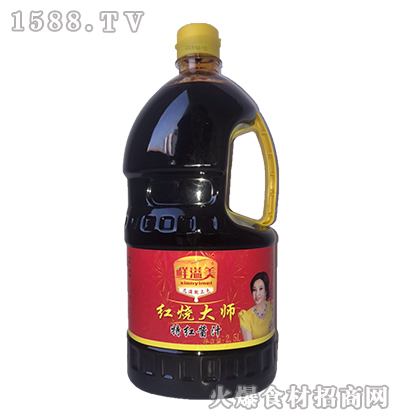我要代理
•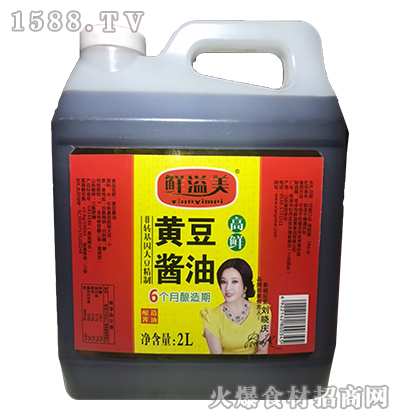我要代理
•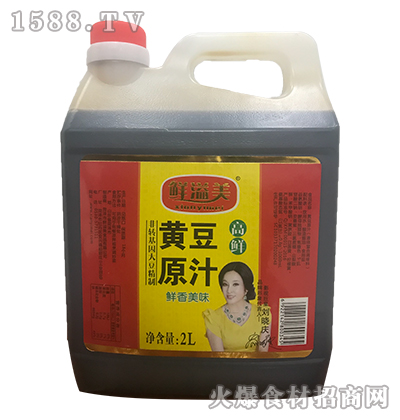我要代理
•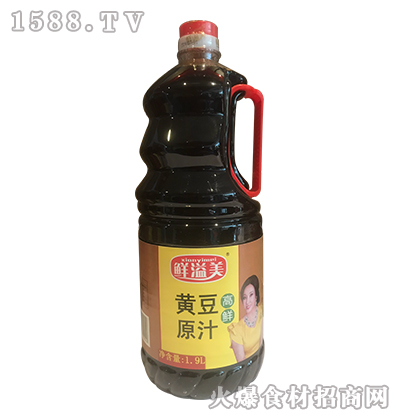我要代理
•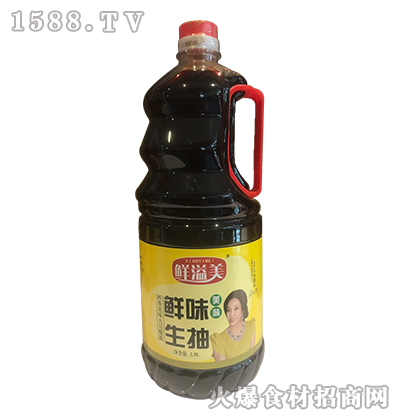我要代理
•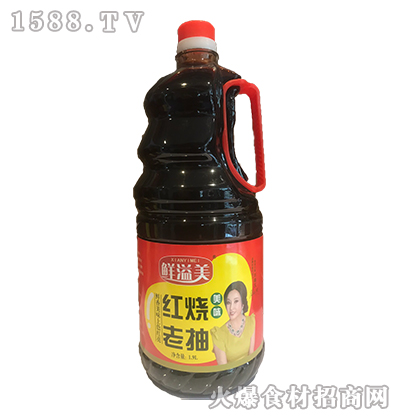我要代理
•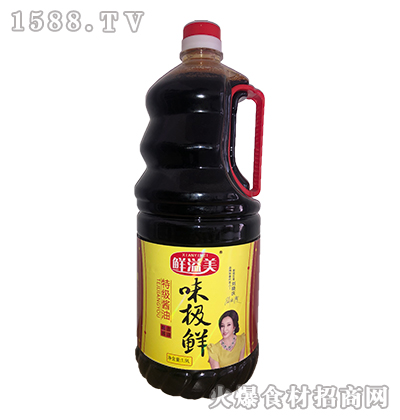我要代理
•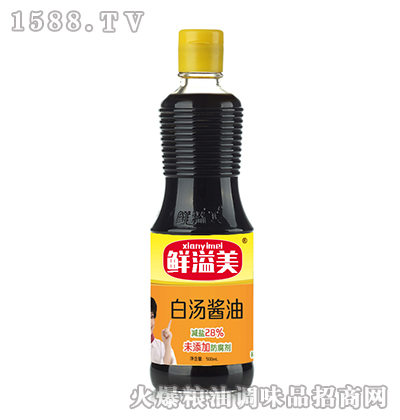我要代理
•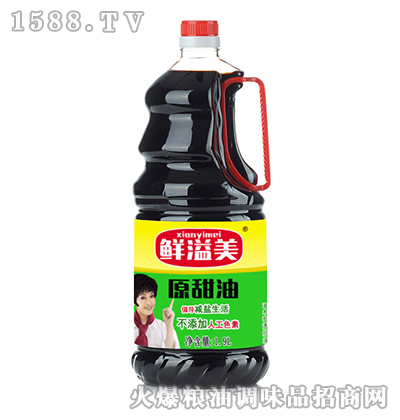我要代理
•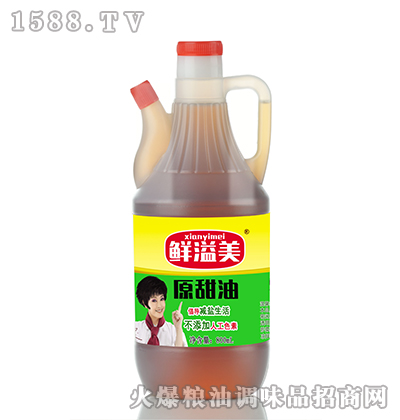我要代理
•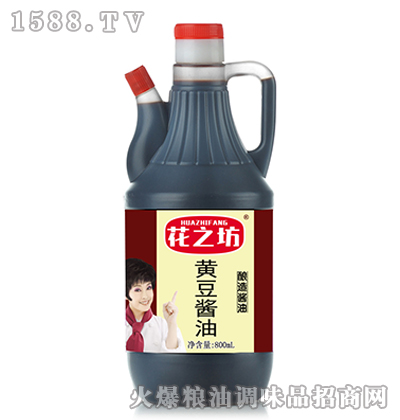我要代理
•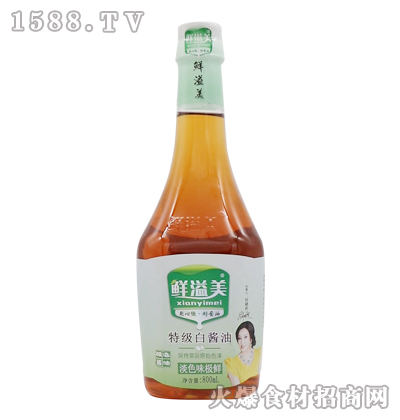我要代理
•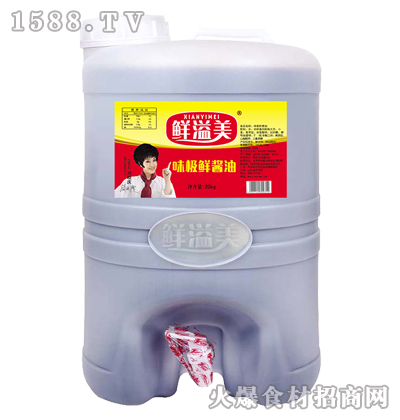我要代理
•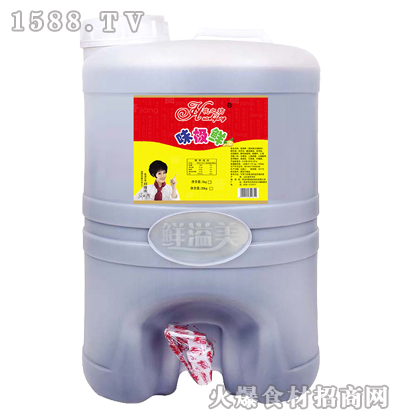我要代理
•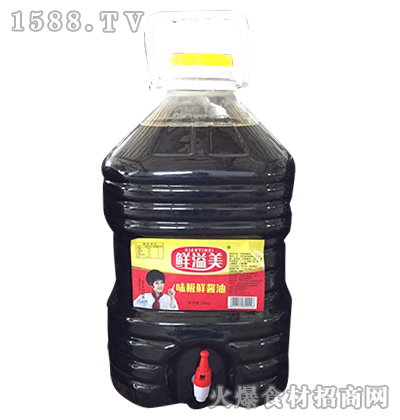我要代理
•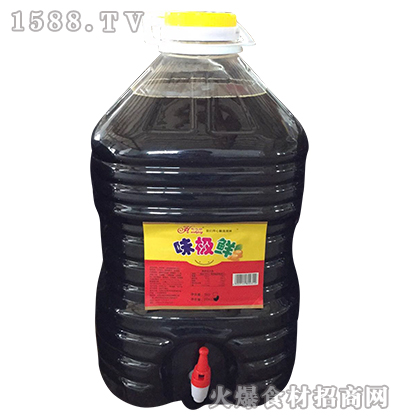我要代理
•我要代理
•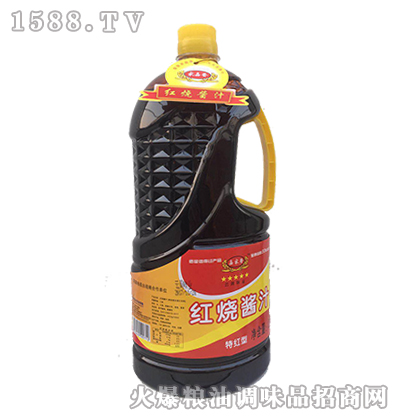我要代理
•我要代理
•我要代理
•我要代理
•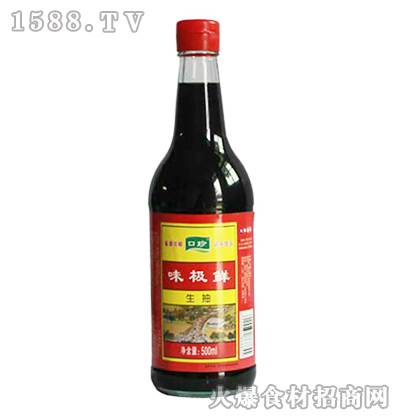我要代理
•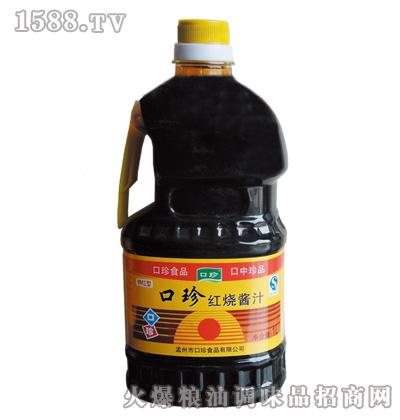我要代理
•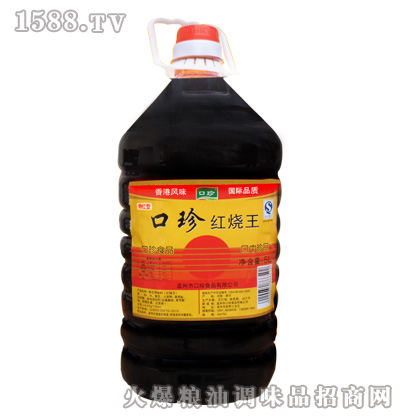我要代理
•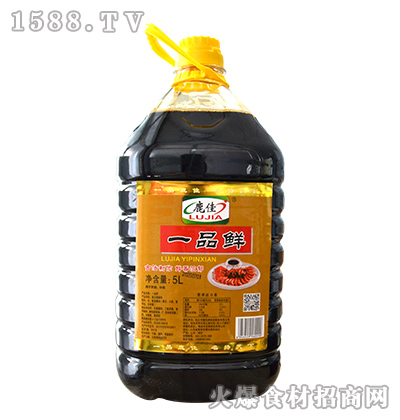我要代理
•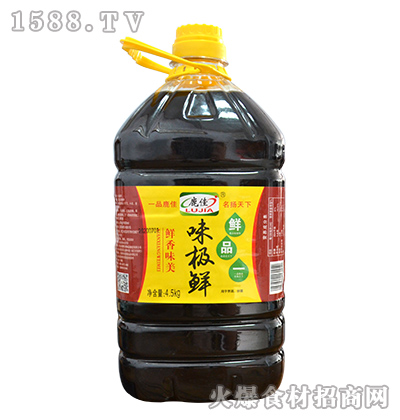我要代理
•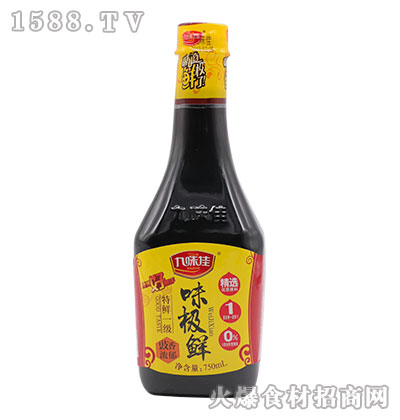我要代理
•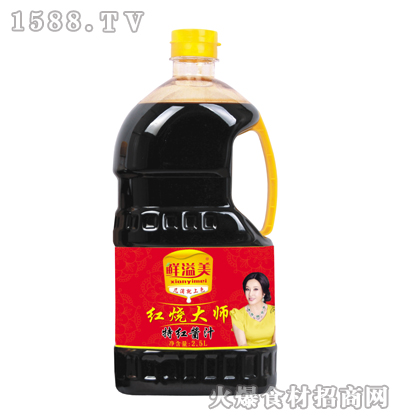我要代理
•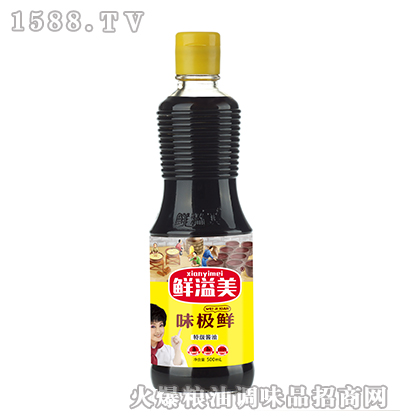我要代理
•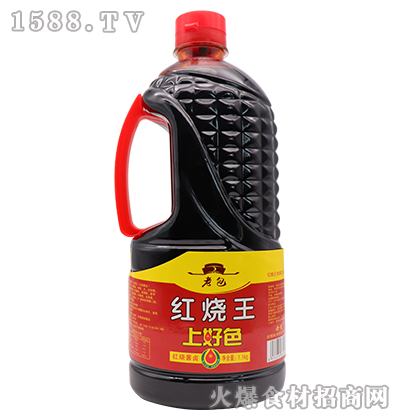我要代理
•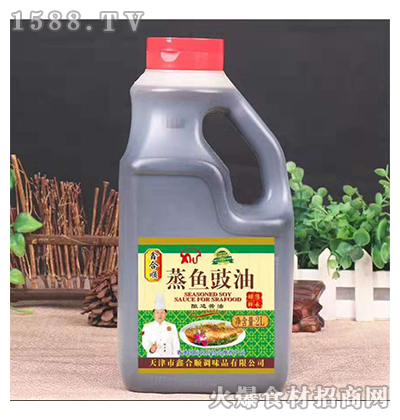我要代理
•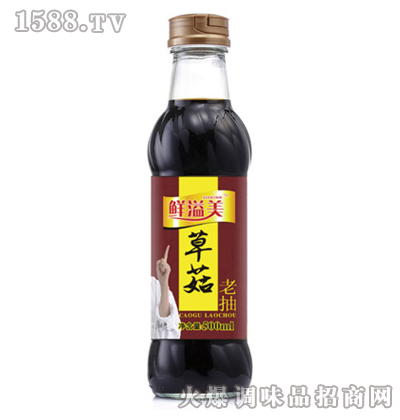我要代理
•我要代理
•我要代理
•我要代理
•我要代理
•我要代理
•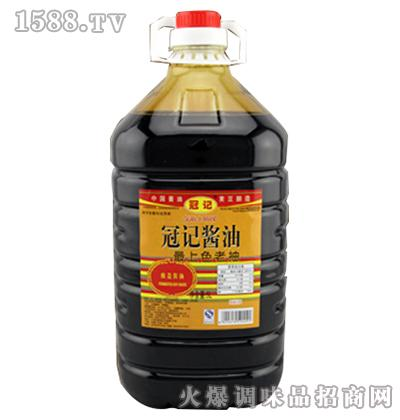我要代理
•我要代理
•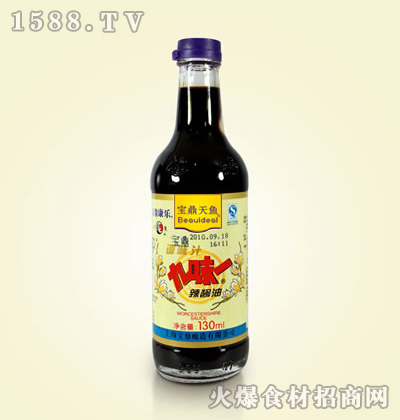我要代理
•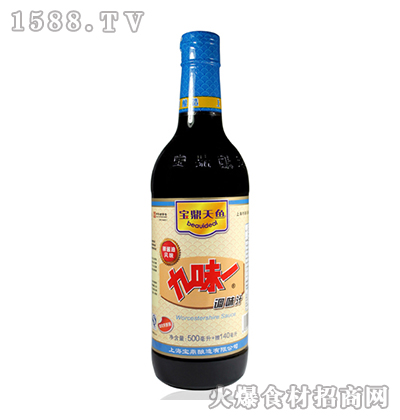我要代理
•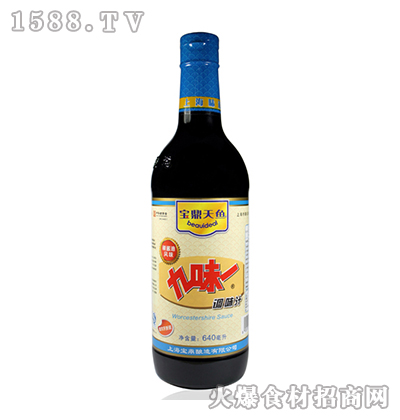我要代理
•我要代理
•我要代理
•我要代理
•我要代理
•我要代理
•我要代理
•我要代理
•我要代理
•我要代理
•我要代理
•我要代理
•我要代理
•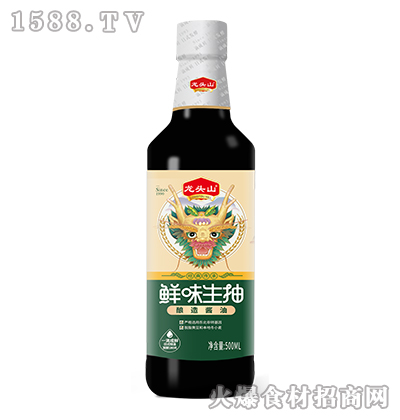我要代理
•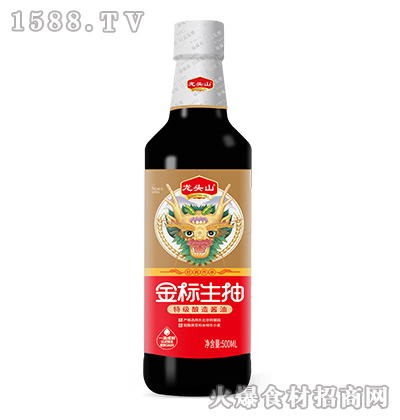我要代理